# Volume and Mass Flow Calculations for Gases

Many times it is necessary to calculate either mass flow or volumetric flow for your application. This same calculation method can also be used to determine the volume at some other set of conditions. To convert mass flow to volume, use the following equation: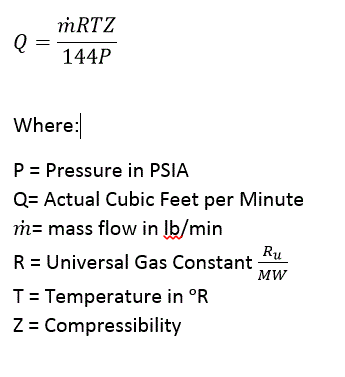Where: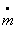= mass flow in lbs/min
R = Universal Gas Flow Constant (1545 ftlbf/(lbmol)(°R)) divided by M.W.
T = Gas Temperature in °R (°F + 460)
Z = Compressibility Factor assumed at 1.0 for pressures below 50 psig
P = Gas Pressure in psia
Q = Volumetric Flow in CFM (Cubic Feet per Minute)
As an example, let’ss assume that we have dry air flowing at 100 lb/min, 200°F and 24.7 psia. We will assume a molecular weight (M.W.) of 28.964 lb/lbmol. Since we have low pressure, we will assume that compressibility is 1.0.

Substitute the numbers and we have the following: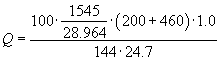This can be simplified to: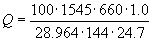The final answer is 989.8 cfm. This could also be called ACFM (Actual Cubic Feet per Minute).

One could also transform this equation to determine mass flow when a volumetric flow is known. To calculate mass flow in lbs/min when volume in cfm (cubic feet per minute) is known, use the following: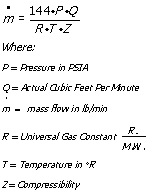Let’s calculate the mass flow of air when a value of 1000 SCFM (Standard Cubic Feet per Minute) is used. To determine the operating parameters, standard conditions must be provided. The most common standard conditions for air is the CAGI or ASME standard which is 14.7 psia (pressure), 68°F and 36% Relative Humidity.

To use our equation, we must first determine the molecular of air at 36% relative humidity. One method that could be used, is to determine the specific gravity of air at 36% relative humidity.

The Specific Gravity of air may be calculated as follows:Where:
SG = Specific Gravity (a number equal to or smaller then 1)
RHa = Relative Humidity at actual conditions (in % ie. 0.36)
PVa = Vapor pressure of water at the actual temperature (psia)
Pb = Barometric Pressure at site location (psia)

If we substitute our conditions into the equation, it looks like this: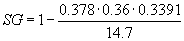This equation simplifies to 0.997. We then multiply the molecular weight of dry air by the specific gravity to get the molecular weight at our conditions of 14.7 psia, 68°F and 36% Relative Humidity.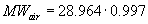Therefore, the molecular weight of air at standard conditions is 28.873 lb/ lbmol.

Now that we have our molecular weight, we can calculate our mass flow as follows: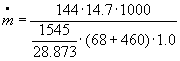Therefore 1000 scfm translates to 74.92 lbs/min of air.

This means that if you have a process that requires 74.92 lbs/min of air, volume must be corrected for any conditions other then standard conditions. Elevation, ambient temperature and relative humidity will all affect your mass flow. Typically a blower is sized for the worst case conditions to insure that enough mass is delivered to the process.

### SCFM (Standard CFM) vs. ACFM (Actual CFM)

Calculation Method to convert SCFM (Standard Cubic Feet per Minute) to ACFM (Actual Cubic Feet per Minute). The calculation is based on standard conditions of 68°F, 36% relative humidity (RH) and 14.7 PSIA.